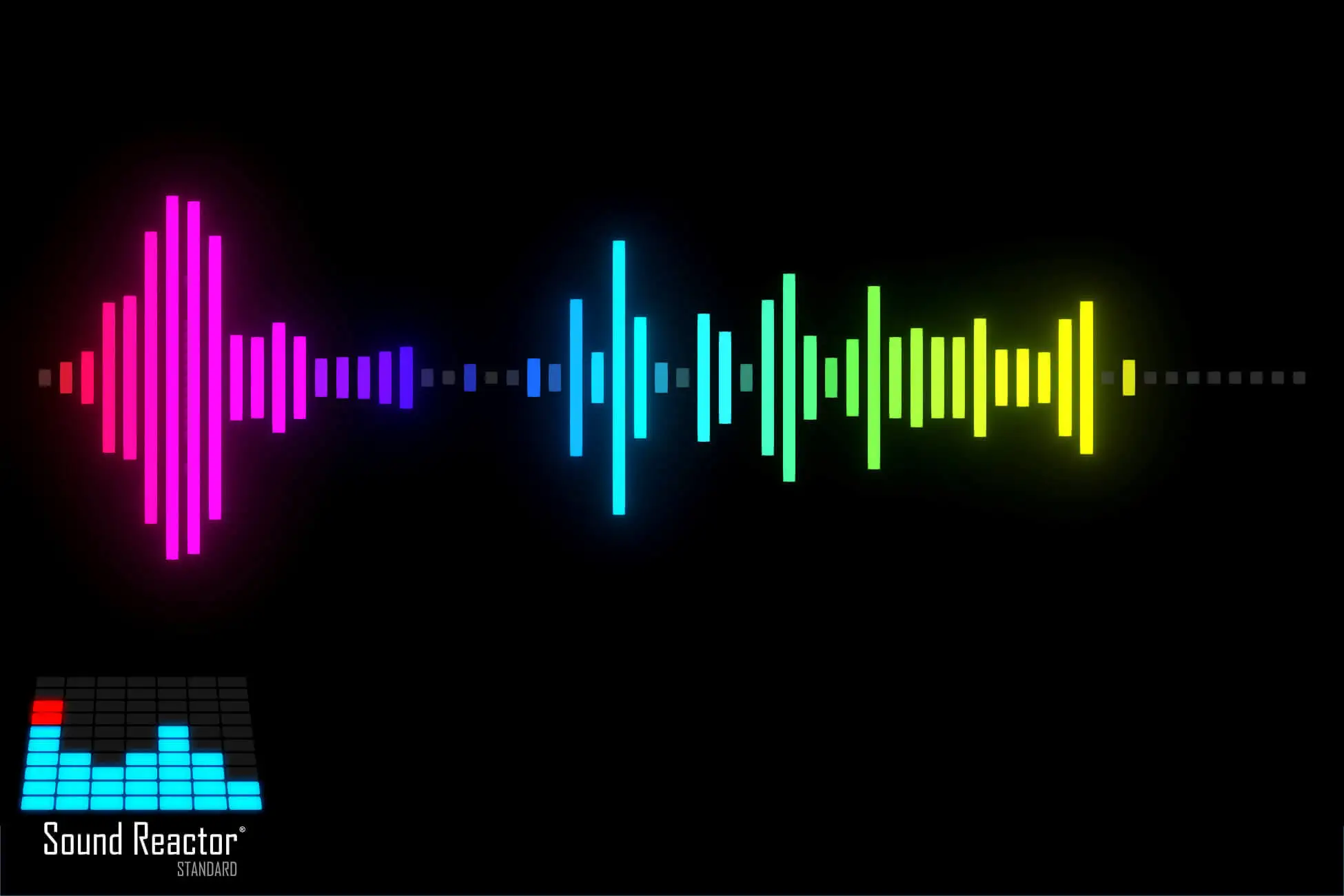1. 每一帧去取音频数据 查看Unity的 AudioSource API，我们发现有一个 GetOutputData 方法，获取音频输出数据，返回类型是一个 float 数组。

2. 取到音频数据后，将数据进行标准化处理 就是找出音频数据中最大的值，然后用每一个值，除以最大值，就可以将所有音频数据映射为 0 到 1 之间的数值大小，返回新的 float 数组。

3. 有了音频数据大小，我们就可以映射为UI的条形图的大小 假设我们限定条状UI的大小最小为10，最大为200，再配合上面取到的音频数据，就可以设定UI的大小了。假设有10个条，那只需要使 float 数组中前10个数值就行。

using UnityEngine;
using System.Collections;
using System.Collections.Generic;
using UnityEngine.UI;

public class AudioV : MonoBehaviour
{
public AudioSource audioSource;
public float minSize = 10;
public float maxSize = 200;
public float lerpSpeed = 20.0f; // 用于控制变化速度
private List<RectTransform> barList = new List<RectTransform>();

// 注意，采样数组的大小必须为2的次方
// 另外，采样数据的个数，一定要 >= 条形UI的数量
private float[] sampleData = new float;

private void Start()
{
// 查找所有的子物体，就是我们要用来显示音频数据的条形UI
RectTransform[] childs = GetComponentsInChildren<RectTransform>();
for (int i = 1; i < childs.Length; ++i)
{
}
}

/// <summary>
/// 进行标准化数据，将数据映射为 0 到 1 之间的数值
/// </summary>
/// <param name="input"></param>
/// <returns></returns>
private float[] NormalizeData(float[] input)
{
float[] output = new float[input.Length];
float max = 0;
float min = 0;
for (int i = 0; i < input.Length; i++)
{
max = Mathf.Max(max, input[i]);
min = Mathf.Min(min, input[i]);
}

float len = max - min;

for (int i = 0; i < input.Length; i++)
{
if (len <= 0)
{
output[i] = 0;
}
else
{
output[i] = (input[i] - min) / len;
}
}

return output;
}

void Update()
{
float[] normalizedData = null;

// 获取原始采样数据
audioSource.GetOutputData(sampleData, 0);

// 进行标准化处理
normalizedData = NormalizeData(sampleData);

for (int i = 0; i < barList.Count; ++i)
{
float newHeight = minSize + (maxSize - minSize) * normalizedData[i];
float currHeight = Mathf.Lerp(barList[i].sizeDelta.y, newHeight, Time.deltaTime * lerpSpeed);

barList[i].SetSizeWithCurrentAnchors(RectTransform.Axis.Vertical, currHeight);
}
}
}